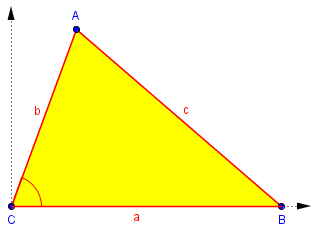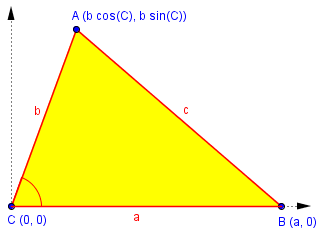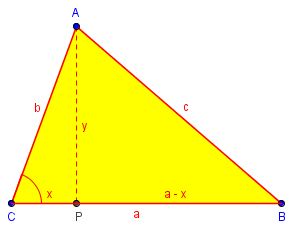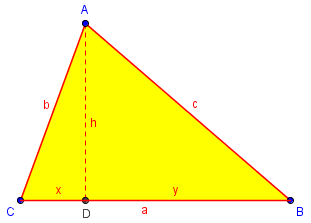# Proving the Law of Cosines

Last week we looked at several proofs of the Law of Sines. Here we will see a couple proofs of the Law of Cosines; they are more or less equivalent, but take different perspectives – even one from before trigonometry and algebra were invented!

## Proof using coordinates

First, here is a question we looked at last time asking about both the Law of Sines and the Law of Cosines; this time we’ll see the answer to the latter part:

Derivation of Law of Sines and Cosines

How do you derive the law of sines and the law of cosines?

Generally, there are several ways to prove the Law of Sines and the Law of Cosines, but I will provide one of each here: Let ABC be a triangle with angles A, B, C and sides a, b, c, such that angle A subtends side a, etc. ...Theorem (Law of Cosines).  c^2 = a^2 + b^2 - 2ab Cos[C].

Proof. Place triangle ABC on a Cartesian coordinate system such that angle C is at the origin and length a lies on the x-axis. Then length b is on the other ray from the origin. We can easily identify the coordinates of two of the vertices: Vertex C lies at (0,0), and vertex B lies at (a,0).  We must identify vertex A, which lies somewhere in quadrant I or II (since angle C < 180 degrees). But the x-coordinate of A is b Cos[C], as can be seen by drawing the perpendicular from A to the x-axis. Similarly, the y-coordinate is b Sin[C]. Hence A lies at (b Cos[C], b Sin[C]).Therefore, the length of c is given by the distance between A and B, which is by the distance formula

c = Sqrt[(a - b Cos[C])^2 + (b Sin[C])^2]

or upon squaring both sides,

c^2 = (a - b Cos[C])^2 + (b Sin[C])^2
= a^2 - 2ab Cos[C] + b^2 Cos[C]^2 + b^2 Sin[C]^2
= a^2 + b^2 (Cos[C]^2 + Sin[C]^2) - 2ab Cos[C].

But Cos[C]^2 + Sin[C]^2 = 1 for any angle C, and therefore

c^2 = a^2 + b^2 - 2ab Cos[C].

So the work is mostly algebra, with a trig identity thrown in. In fact, we used the Pythagorean Theorem at least twice, first in the form of the distance formula, and again in the form of the Pythagorean identity, $$\sin^2 \theta + \cos^2 \theta = 1$$.

I hope that was understandable. To really get a feel for the proofs, draw out the diagrams. Clearly, the Law of Cosines is a more difficult proof, but it is really a generalization of the Pythagorean Theorem, since in the right-angle case (C = 90), Cos[C] = 0 and we obtain c^2 = a^2 + b^2. This is why we often see the Law of Cosines written as c^2 = ... instead of the equally valid form

a^2 = b^2 + c^2 - 2bc Cos[A].

Applying the Law of Cosines to each of the three angles, we have the three forms

$$a^2 = b^2 + c^2 – 2bc\cos(A)$$

$$b^2 = a^2 + c^2 – 2ac\cos(B)$$

$$c^2 = a^2 + b^2 – 2ab\cos(C)$$

A virtually identical proof is found in this page we also looked at last time:

Proving Laws of Sines, Cosines

## Proof using the Pythagorean Theorem

The next question was from a student who just guessed that there should be a way to modify the Pythagorean Theorem to work with non-right triangles; that is just what the Law of Cosines is. But since Brooke apparently does not know trigonometry yet, a mostly geometrical answer seemed appropriate.

Deriving the Law of Cosines

I wonder if the Pythagorean Theorem will work with a non-right triangle and how to go about proving it.

This is a good question, and it shows good thinking. The Pythagorean Theorem will not work for triangles that are not right triangles.  When you have a triangle which is not right, the closest analogue is something called the Law of Cosines.

If the sides of a triangle are labeled a, b, and c, and the angles opposite them A, B, and C, respectively, then:

c^2 = a^2 + b^2 - 2*a*b*cos(C)

Here "cos(C)" means the cosine of the angle C. If the triangle is a right triangle with right angle C, then the cosine of C, that is, the cosine of 90 degrees, is zero, so you get c^2 = a^2 + b^2, the Pythagorean Theorem.

So the Pythagorean Theorem can be seen as a special case of the Law of Cosines.

If the angle is other than 90 degrees, however, the cosine of C is not zero. For example, if the angle C is 60 degrees, its cosine is 1/2, and you get the equation c^2 = a^2 +b^2 - a*b. If C is 120 degrees, its cosine is -1/2, and you get the equation c^2 = a^2 + b^2 + a*b.


You may find it interesting to see what happens when angle C is 0° or 180°! These are not literally triangles (they can be called degenerate triangles), but the formula still works: it becomes mere addition or subtraction of lengths.

Now he gives an algebraic proof similar to the one above, but starting with geometry rather than coordinates, and avoiding trigonometry until the last step:

You will learn about cosines and prove the Law of Cosines when you study trigonometry. The proof depends on the Pythagorean Theorem, strangely enough! Draw triangle ABC with sides a, b, and c, as above.  Drop a perpendicular from A to BC, meeting it at point P. Let the length AP be y, and the length CP be x. Then BP = a-x. Then apply the Pythagorean Theorem to the two triangles ACP and ABP:

CP^2 + AP^2 = AC^2   or   x^2 + y^2 = b^2
BP^2 + AP^2 = AB^2   or   (a-x)^2 + y^2 = c^2(I’ve swapped the names of x and y from the original, to increase the similarity to our coordinate proof above.)

Now subtract the first equation from the second, to eliminate y:

(a-x)^2 - x^2 = c^2 - b^2
a^2 - 2*a*x + x^2 - x^2 = c^2 - b^2
a^2 - 2*a*x = c^2 - b^2
c^2 = a^2 + b^2 - 2*a*b*(x/b)

This is the non-trigonometric version of the Law of Cosines.

Now x/b is just the cosine of angle C (if you don't know this, trust me), so:

c^2 = a^2 + b^2 - 2*a*b*cos(C)

Observe that C is a right angle if and only if x = 0 and points P and C coincide.

## The Law of Cosines in Euclid

Here is a question from 2006 that was not archived:

Hi, I'm a student at the University of Minnesota Twin Cities Campus, and I'm an Actuarial Science major.  I'm writing an article, and it involves stating the proofs of the Law of Sines and the Law of Cosines.  I found the proofs very easily by researching on the internet, but I would like to add one possible sentence on who first proved the Law of Sines and the Law of Cosines if possible, just to make it a bit more interesting.  If you know, please let me know.  Thank you very much.

I replied,

The Law of Cosines, at least, can be found in Euclid -- which was before cosines were defined! See this page:

http://aleph0.clarku.edu/~djoyce/java/elements/bookII/propII12.html

For another discussion, see

http://www.cut-the-knot.org/pythagoras/cosine2.shtml

I don't find that the Law of Sines is found in Euclid, but it can be proved easily from Euclid, and in some form was proved quite early.

For more details, see

http://en.wikipedia.org/wiki/Law_of_cosines
http://en.wikipedia.org/wiki/Law_of_sines

The latter, unfortunately, doesn't mention history, as the former does.

The Cut-the-Knot page includes several proofs, as does Wikipedia.

Euclid has two propositions (one applying to an obtuse triangle, the other to acute), because negative numbers were not acceptable then (and the theorems don’t use numbers in the first place, but lengths!). Neither trigonometric functions nor algebraic concepts existed yet, so everything had to be expressed in terms of geometry. This makes for a very interesting perspective on the proof!

Let’s just look at the acute case:

Euclid’s Elements: Proposition II.13

In acute-angled triangles the square on the side opposite the acute angle is less than the sum of the squares on the sides containing the acute angle by twice the rectangle contained by one of the sides about the acute angle, namely that on which the perpendicular falls, and the straight line cut off within by the perpendicular towards the acute angle.

If you never realized how much easier algebraic notation makes things, now you know!

If we label the triangle as in our previous figures, we have this:The theorem says, in the geometric language Euclid had to use, that:

The square on the side opposite the acute angle [ $$c^2$$ ] is less than the sum of the squares on the sides containing the acute angle [ $$a^2 + b^2$$ ] by twice the rectangle contained by one of the sides about the acute angle, namely that on which the perpendicular falls [a], and the straight line cut off within by the perpendicular towards the acute angle [x, so the rectangle is $$2ax$$].

That is, $$c^2 = a^2 + b^2 – 2ax$$

Since $$x = b\cos(C)$$, this is exactly the Law of Cosines, without explicit mention of cosines.

I won’t quote the proof, which uses different labels than mine; but putting it in algebraic terms, it amounts to this:

From a previous theorem (Proposition II.7), $$a^2 + x^2 = 2ax + y^2$$

[This amounts to our algebraic fact that $$y^2 = (a – x)^2 = a^2 – 2ax + x^2$$.]

Adding $$h^2$$ to each side, $$a^2 + x^2 + h^2 = 2ax + y^2 + h^2$$

But from the two right triangles $$\triangle ACD$$ and $$\triangle ABD$$, $$x^2 + h^2 = b^2$$, and $$y^2 + h^2 = c^2$$. So our equation becomes $$a^2 + b^2 = 2ax + c^2$$

Rearranging, we have our result: $$c^2 = a^2 + b^2 – 2ax$$

Again, we have a proof that is substantially the same as our others – but this one is more than 2000 years older!

### 1 thought on “Proving the Law of Cosines”

1.Thank you this was very clear

This site uses Akismet to reduce spam. Learn how your comment data is processed.